Most Affordable JEE | NEET | 8,9,10 Preparation by Kota's Top IITian Doctor Faculties

# Biomolecule - JEE Advanced Previous Year Questions with Solutions`
JEE Advanced Previous Year Questions of Chemistry with Solutions are available at eSaral. Practicing JEE Advanced Previous Year Papers Questions of Chemistry will help the JEE aspirants in realizing the question pattern as well as help in analyzing weak & strong areas.   Simulator Previous Years JEE Advanced Questions
Q. The correct statement(s) about the following sugars X and Y is(are)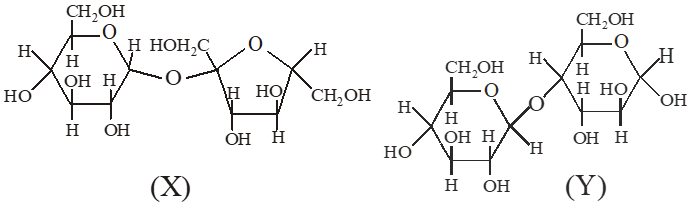(A) X is a reducing sugar and Y is a non‑reducing sugar (B) X is a non‑reducing sugar and Y is a reducing sugar (C) The glucosidic linkages in X and Y are $\alpha$ and $\beta$ , respectively. (D) The glucosidic linkages in X and Y are $\beta$ and $\alpha$ , respectively. [JEE 2009]
Ans. (B,C)
Q. Among cellulose, poly vinyl chloride, nylon and natural rubber, the polymer in which the intermolecular force of attraction is weakest is (A) Nylon (B) Poly (vinyl chloride) (C) Cellulose (D) Natural Rubber [JEE 2009]
Ans. (D) Natural rubber is elastomer and has weak vander waal force of attraction and the unit (monomer) is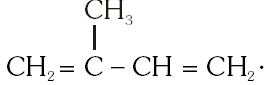Q. The following carbohydrate is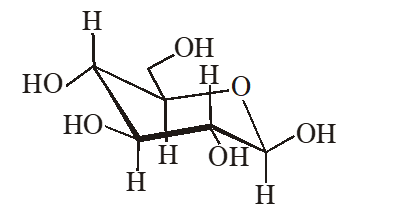(A) a ketohexose (B) an aldohexose (C) an $\alpha$-furanose (D) an $\alpha$-pyranose [JEE 2011]
Ans. (B)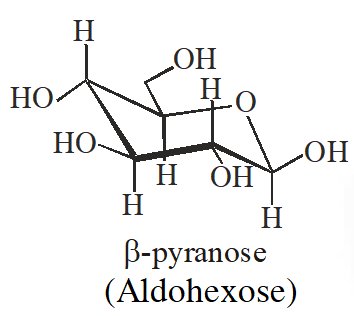Q. The correct statement about the following disaccharide is –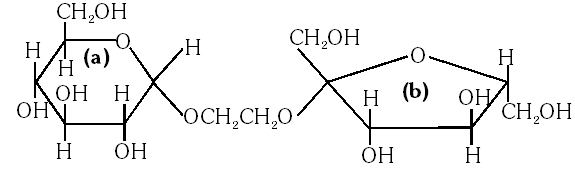(A) Ring (a) is pyranose with a–glycosidic link (B) Ring (a) is furanose with a–glycosidic link (C) Ring (b) is furanose with a–glycosidic link (D) Ring (b) is pyranose with b–glycosidic link [IIT-2010]
Ans. (A) The ring is pyranose with glycosidic linkage which means the oxide linkage which connect the monosaccharide units in polysaccharides.
Q. The total number of basic groups in the following form of lysine is : (A) 3             (B) 0             (C) 2             (D) 1 [IIT-2010]
Ans. (C) The two basic groups are $\mathrm{NH}_{2}$ and $\mathrm{COO}^{-}$ groups.
Q. The major product of the following reaction is (A) a hemiacetal          (B) an acetal           (C) an ether            (D) an ester [JEE 2011]
Ans. (B)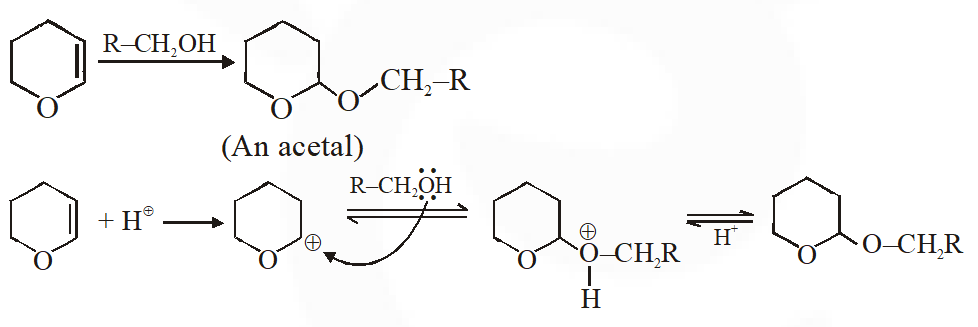Q. Amongst the compounds given, the one that would form a brilliant coloured dye on treatment with $\mathrm{NaNO}_{2}$ in dil. HCl followed by addition to an alkaline solution of $\beta$ naphthol is –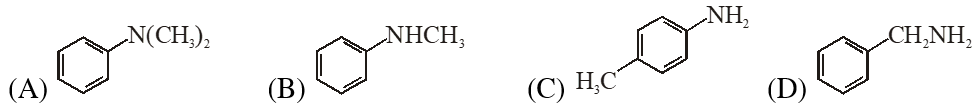[JEE 2011]
Ans. (C)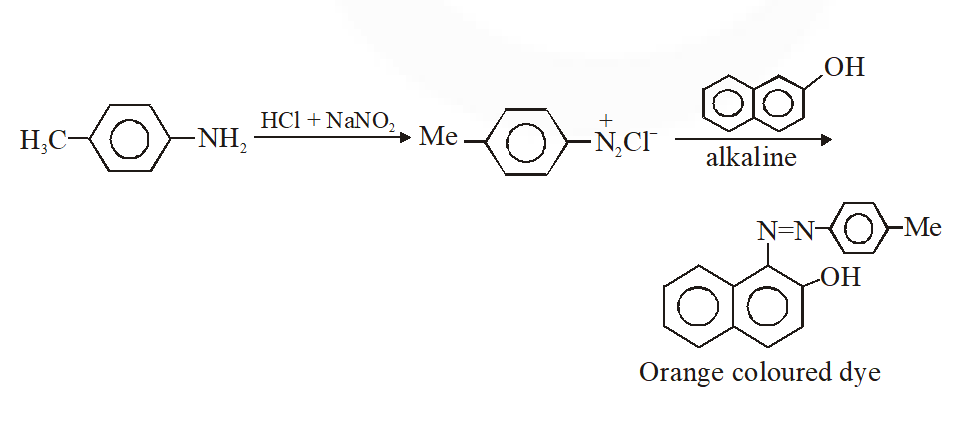Q. The correct functional group X and the reagent/reaction conditions Y in the following scheme are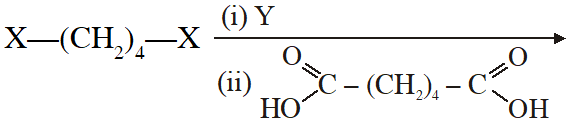condensation polymer (A) $\mathrm{X}=\mathrm{COOCH}_{3}, \mathrm{Y}=\mathrm{H}_{2} / \mathrm{Ni} / \mathrm{heat}$ (B) $\mathrm{X}=\mathrm{CONH}_{2}, \mathrm{Y}=\mathrm{H}_{2} / \mathrm{Ni} / \mathrm{heat}$ (C) $\mathrm{X}=\mathrm{CONH}_{2}, \mathrm{Y}=\mathrm{Br}_{2} / \mathrm{NaOH}$ (D) $\mathrm{X}=\mathrm{CN}, \mathrm{Y}=\mathrm{H}_{2} / \mathrm{Ni} / \mathrm{heat}$ [JEE 2011]
Ans. (A,B,C,D)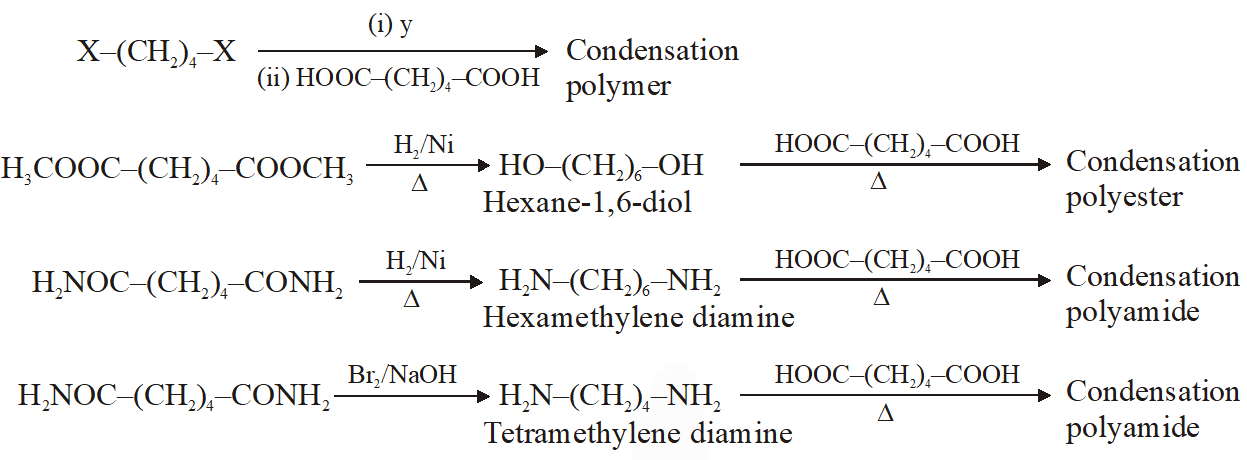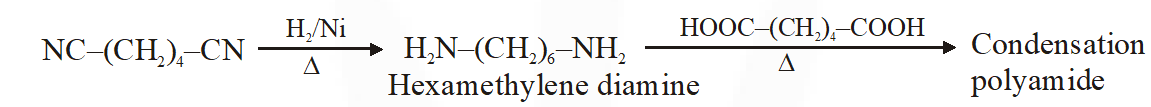Q. The structure of D-(+)-glucose is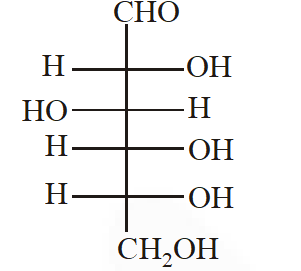The structure of L(–)-glucose is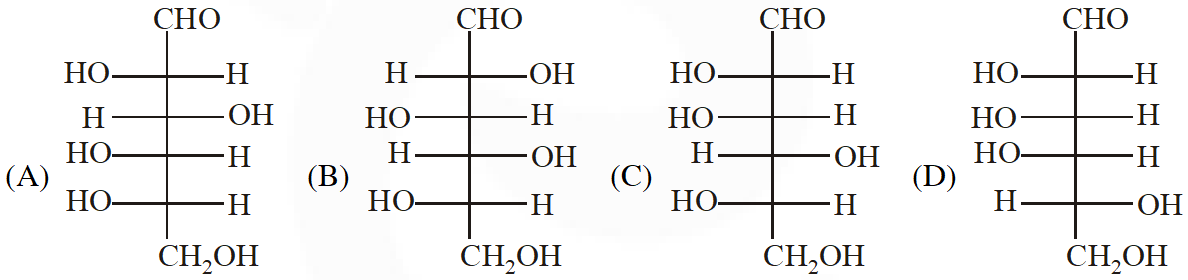[IIT 2011]
Ans. (A) The structure of D(+) glucose is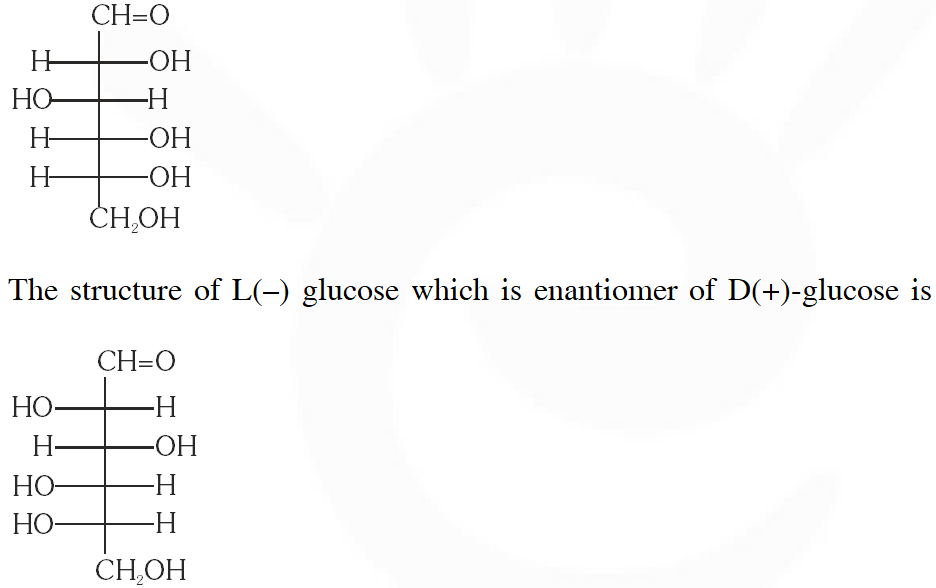Q. A decapeptide (Mol. Wt. 796) on complete hydrolysis gives glycine (Mol. Wt. 75), alanine and phenylalanine. Glycine contributes 47.0% to the total weight of the hydrolysed products. The number of glycine units present in the decapeptide is [JEE 2011]
Ans. 6 No. of peptide linkage $=$ No. of water molecules added for complete hydrolysis. = n – 1 So, number of molecules of $\mathrm{H}_{2} \mathrm{O}$ added $=9$ So total wt. of the product $=$ Mol. wt. of polypeptide $+$ total wt. of $\mathrm{H}_{2} \mathrm{O}$ added. = 796 + (9 × 16) = 796 + 162 = 958 $\therefore$ wt. of glycine obtained $=958 \times \frac{47}{100} \square 450$ No. of units of glycine $=\frac{450}{75}=6$ units
Q. The substitutes $R_{1}$ and $R_{2}$ for nine peptides are listed in the table given below. How many of these peptides are positively charged at $p H=7.0 ?$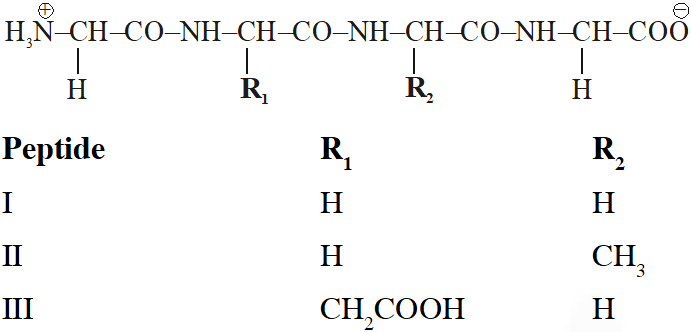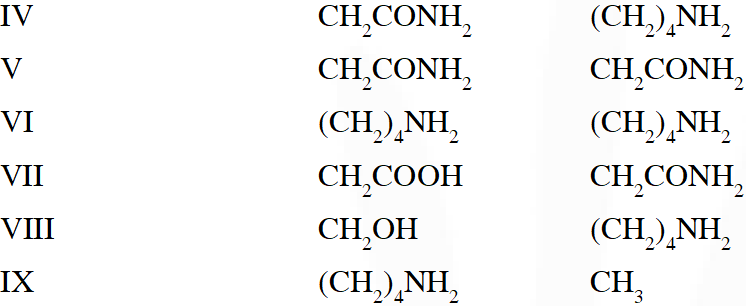[JEE 2012]
Ans. 4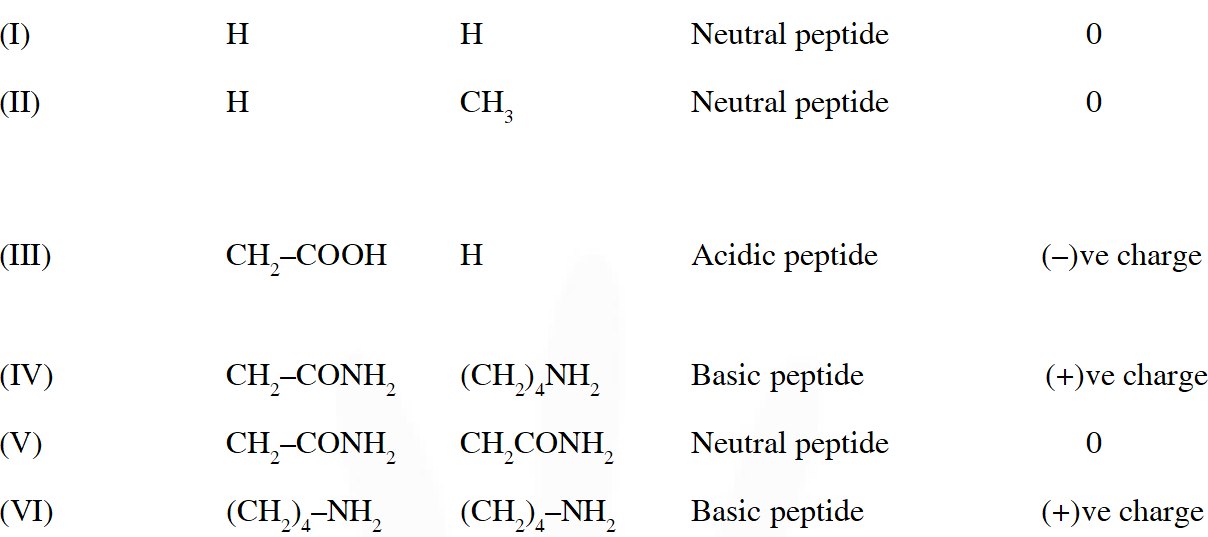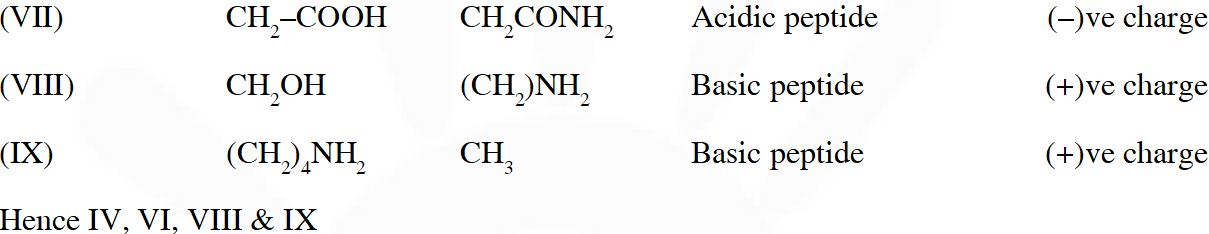Q. When the following aldohexose exists in its d-configuration , the total number of stereoisomers in its pyranose form is –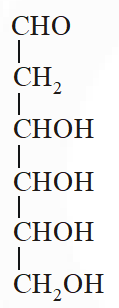[JEE 2012]
Ans. 8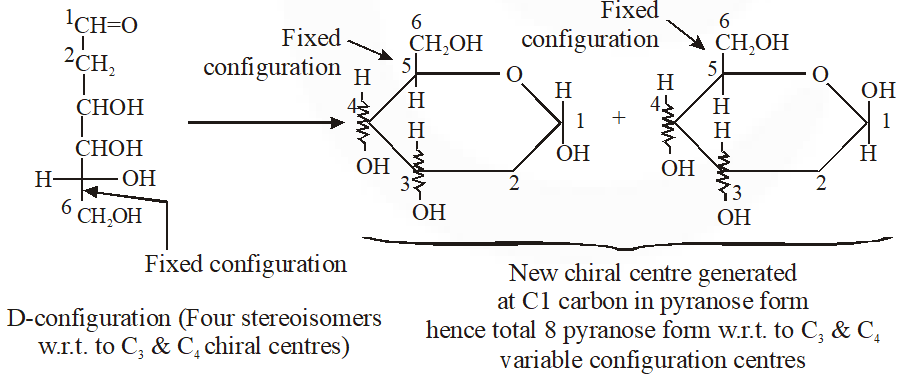Q. A tetrapeptide has –COOH group on alanine. This produces glycine (Gly), valine (Val), phenyl alanine (Phe) and alanine (Ala), on complete hydrolysis. For this tetrapeptide, the number of possible sequences (Primary structures) with –$\mathrm{NH}_{2}$ group attached to a chiral center is [JEE 2013]
Ans. 4 Considering alanin at one end of tetra peptides structure with $\mathrm{CO}_{2} \mathrm{H}$ group, number of possible combination are as follows: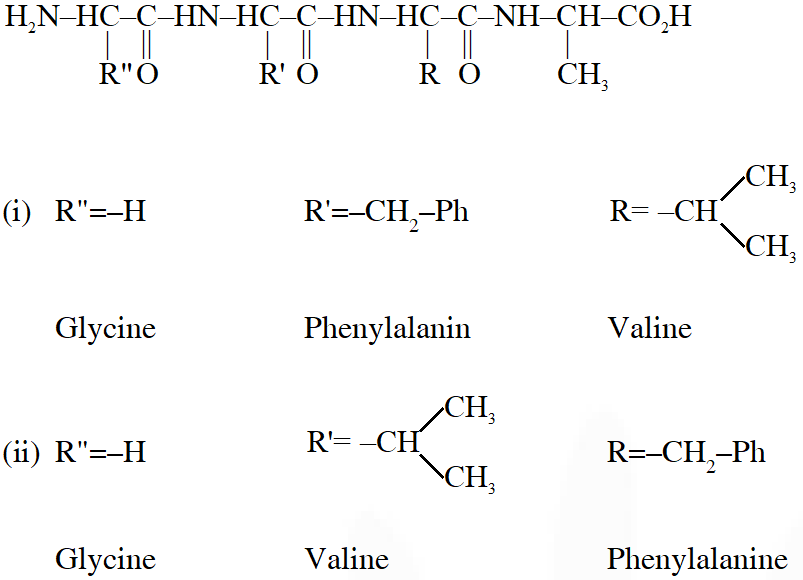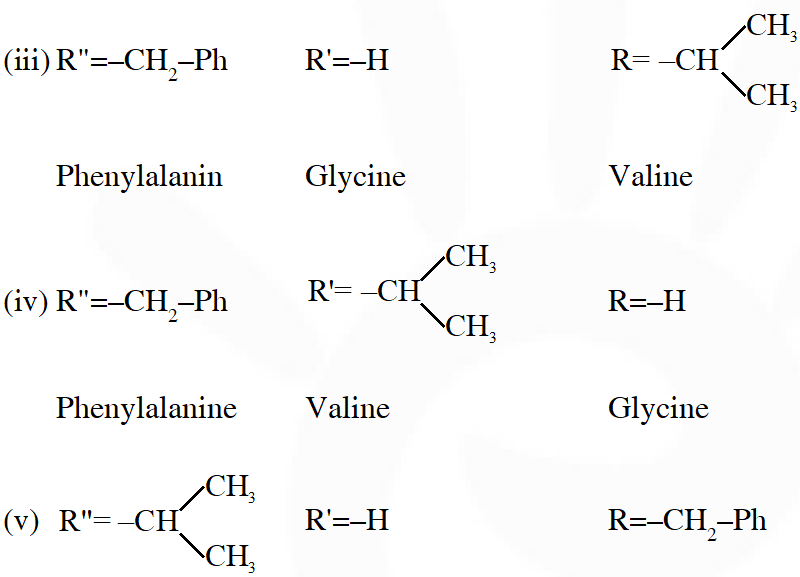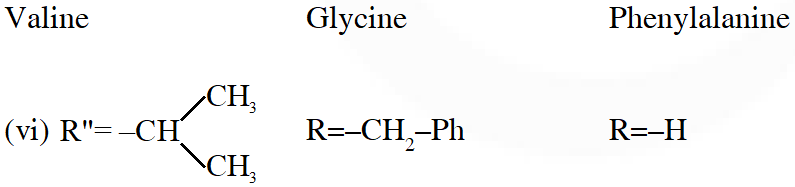(i) $\&$ (ii) can not be the possible because in those combination $-\mathrm{NH}_{2}$ group is not attached to chiral centre. Hence, answer is (4)
Q. The total number of distinct naturally occurring amino acids obtained by complete acidic hydrolysis of the peptide shown below is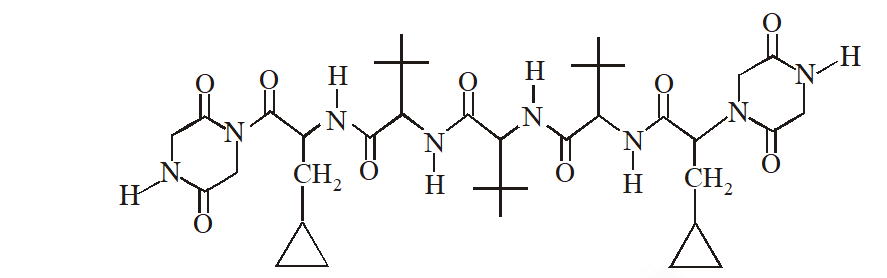[JEE 2014]
Ans. 1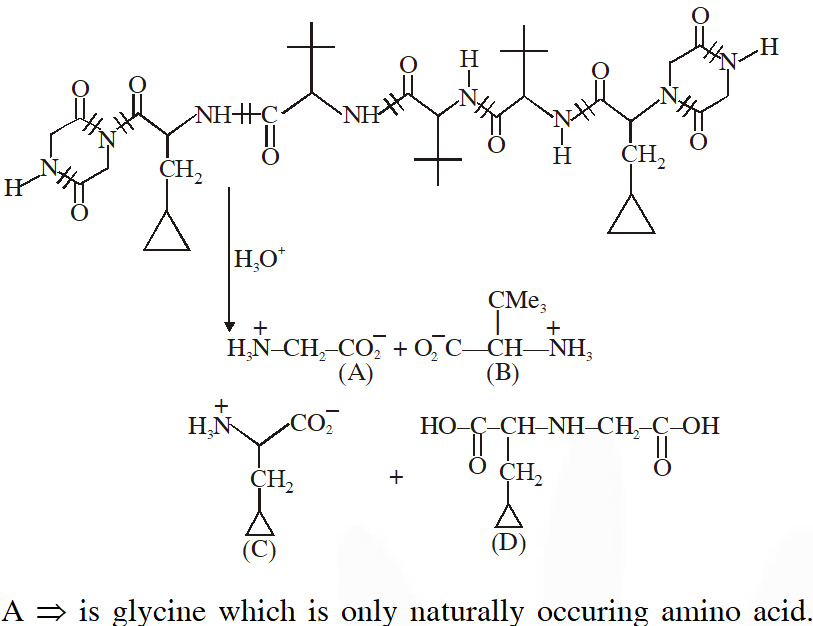Q. Positive Tollen's test is observed for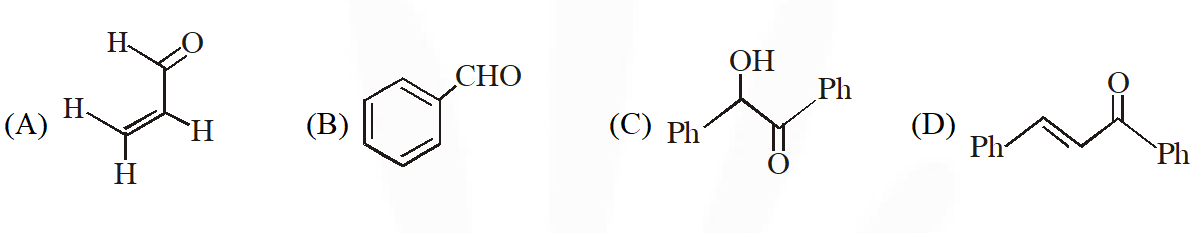[JEE - Adv. 2016]
Ans. (A,B,C) Tollens's test is given by compounds having aldehyde group. Also -hydroxy carbonyl gives positive tollen's test.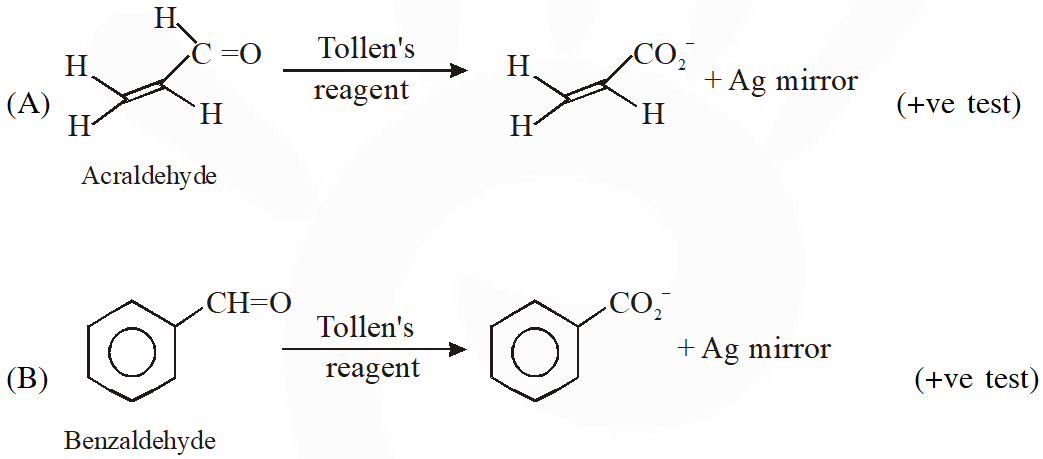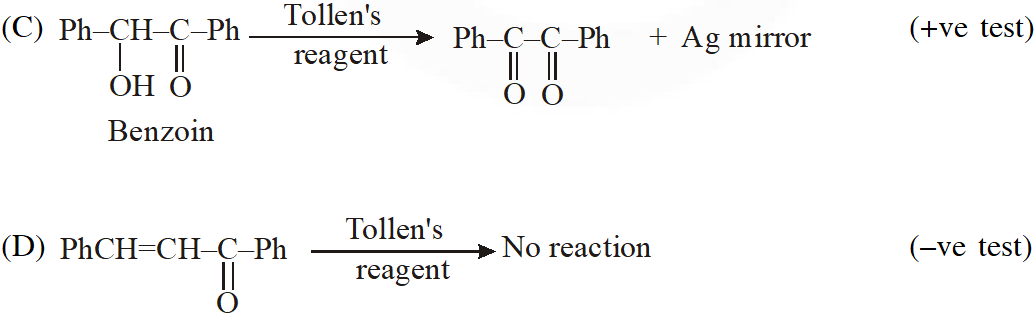Q. For 'invert sugar', the correct statement(s) is (are) (Given : specific rotations of (+)-sucrose, (+)-maltose, L-(–)-glucose and L-(+)-fructose in aqueous solution are $+66^{\circ},+140^{\circ},-52^{\circ}$ and $+92^{\circ}$, respectively) (A) 'invert sugar' is prepared by acid catalyzed hydrolysis of maltose (B) 'invert sugar' is an equimolar mixture of D-(+) glucose and D-(–)-fructose (C) specific rotation of 'invert surgar' is $-20^{\circ}$ (D) on reaction with $\mathrm{Br}_{2}$ water, 'invert sugar' forms saccharic acid as one of the products [JEE - Adv. 2016]
Ans. (B,C) Invert sugar is equailmolar mixture of D-glucose and D-fructose which is obtained by hydrolysis of sucrose Specific rotation of mixture is half of sum of sp. rotation of both components $\frac{+52^{\circ}+\left(-92^{\circ}\right)}{2}$ $=-20^{\circ}$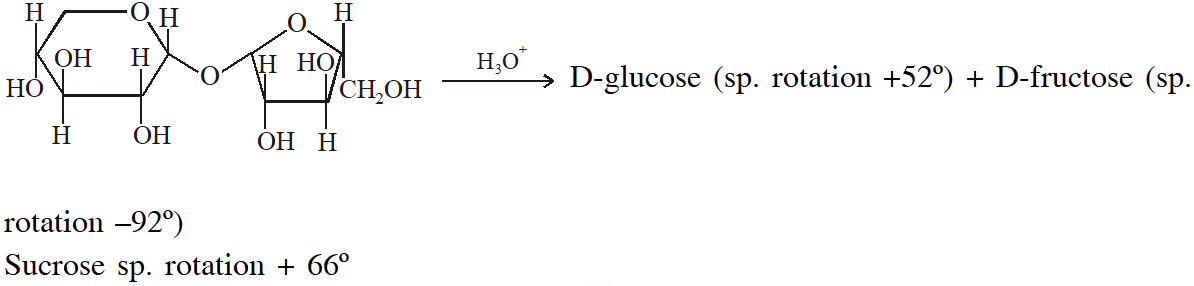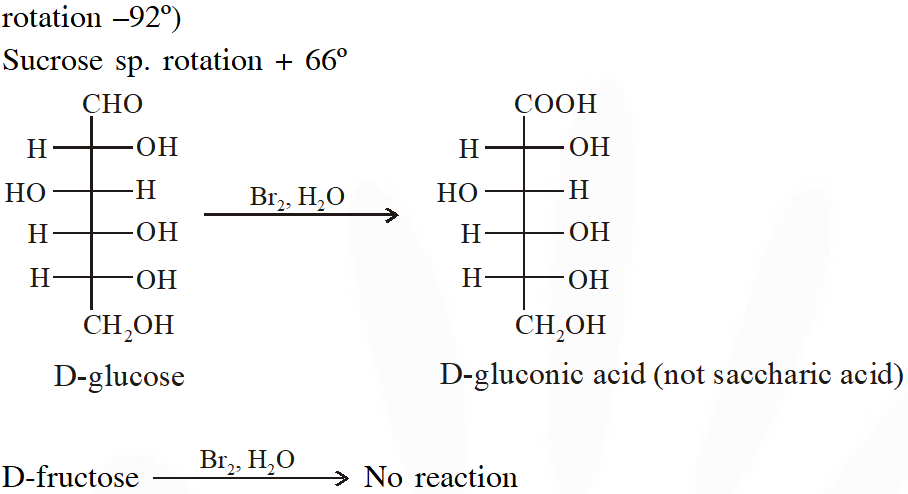Paragraph (q. 17 TO q. 18) Treatment of compound O with $\mathrm{KMnO}_{4} / \mathrm{H}^{+}$ gave P, which on heating with ammonia gave Q. The compound Q on treatment with $\mathrm{Br}_{2} / \mathrm{NaOH}$ produced R. On strong heating, Q gave S, which on further treatmenet with ethyl 2-bromopropanoate in the presence of KOH following by acidification, gave a compound T.
Q. The compound R is :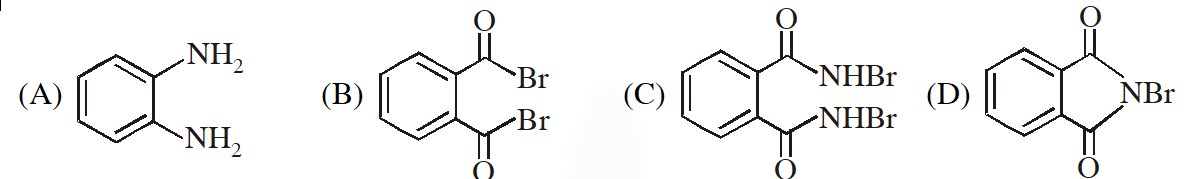[JEE - Adv. 2016]
Ans. (A)
Q. The compound T is : (A) Glycine               (B) Alanine               (C) Valine              (D) Serine [JEE - Adv. 2016]
Ans. (B)
Q. The Fischer presentation of D-glucose is given below.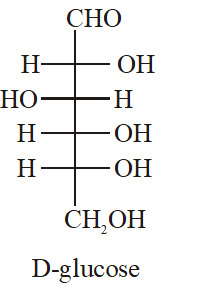The correct structure(s) of $\beta$-glucopyranose is (are) :-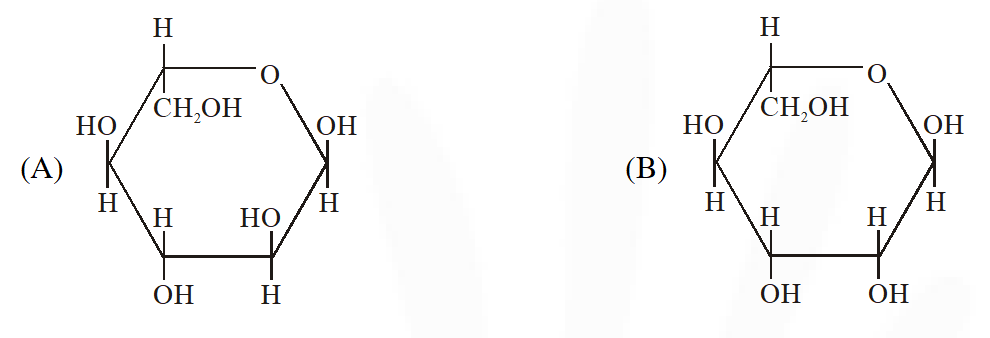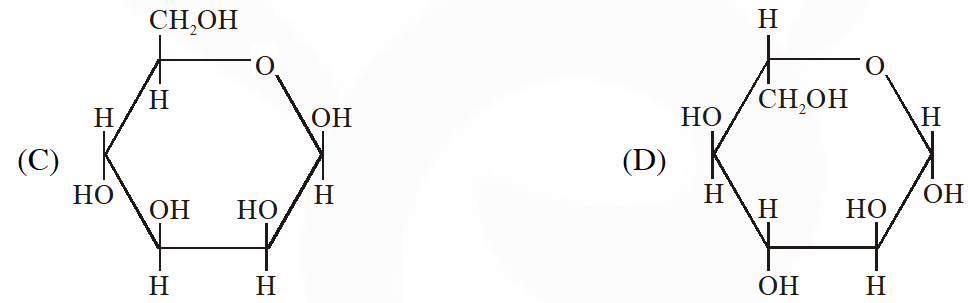[JEE Adv. 2018]
Ans. (D)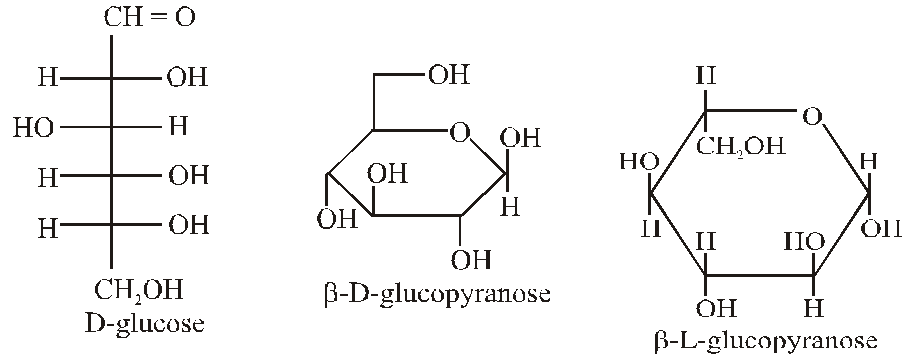Manish
March 7, 2021, 9:30 p.m.
Nice, Thank you.
Keerti
Sept. 26, 2020, 9:20 p.m.
Great Job
Esaaral
Sept. 24, 2020, 11:51 p.m.
Esaral sucks big time
chandana Nayak
Sept. 24, 2020, 2:31 p.m.
Santo
Sept. 24, 2020, 12:34 p.m.
,👍
Vikas
Sept. 20, 2020, 7:11 p.m.
Really awesome
anonymous
Sept. 13, 2020, 6:53 p.m.
very helpful for last minute revision
Suraj
Sept. 13, 2020, 10:27 a.m.
Wowwwwwwwwwwwwwwwwwoooo
Anonymous
Sept. 11, 2020, 12:32 p.m.
Alanine =A., not T
Anonymous
Sept. 11, 2020, 12:26 p.m.
Some solutions r wrong
Rishabh
Sept. 8, 2020, 9:10 p.m.
you guys really do great work, keep it up!!!
Lokesh
July 9, 2020, 9:12 a.m.
good
Piyush Awasthi
May 6, 2020, 2:49 a.m.
In last question L is missing in question , correct it